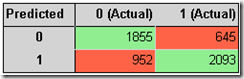## Monday, January 31, 2011

### BOL Community Article about Back-Testing Data Mining Results

SQL Server 2008 | SQL Server 2008 R2

In addition to my blog posts about back-testing Data Mining Results (see part 1, part 2 and part 3) I also wrote a Books Online Community Article that covers the topic at a more detailed level. The article can be downloaded here: http://msdn.microsoft.com/en-us/library/gg557481.aspx. The sample data and solution is available for download. The link is provided in the article.

You will find all the Community Articles for SQL Server 2008 here: http://msdn.microsoft.com/en-us/library/cc872864(SQL.100).aspx

## Sunday, January 16, 2011

### Cumulated Gains Chart and Lift Chart in SSRS

SQL Server 2008 | SQL Server 2008 R2

This post is about reproducing some of the mining charts that are built into Business Intelligence Development Studio using Reporting Services. A common use case might be that you periodically train your model and the results of the training should be published as html reports.

For my example I use the Adventure Works Targeted Mailing mining structure. We want to plot the results from the “TM Decision Tree Model”. First let’s start with the lift chart. In BIDS, the chart for “Bike Buyer=1” looks like this:

In order to reproduce the chart, we’re going to use the SystemGetLiftTable stored function in SSAS. Since we need to do some computations, I prefer to use SQL, so we have to define a linked server first:

@server='SSAS_AW',
@srvproduct='',
@provider='MSOLAP',

The query for loading the results from the lift chart looks like this:

SELECT percentile,
VALUE,
CASE
WHEN percentile >= 100 * CONVERT(FLOAT, totalattributevaluecases) /
totalcases
THEN 100
ELSE percentile / (( CONVERT(FLOAT, totalattributevaluecases) /
totalcases ))
END idealmodel
FROM Openquery(ssas_aw,
'CALL SystemGetLiftTable([TM Decision Tree], 2, ''Bike Buyer'', 1)') AS
derivedtbl_1

For the chart, we use the percentile of the lift table as category group (x-axis) and choose these entries for the values (y-axis):

• Sum(Value)
This is the actual lift curve for our mining model
• Sum(Percentile)
As this is the same value as on the x-axis, this gives the random guess model line (linear)
• Sum(IdealModel)
The case-when-end statement above reflects the curve for the ideal model (linear function from 0 to 1 between 0 and totalattribute/totalcases)

This is how the chart looks like in the SSRS designer:

There are different names for this kind of a chart. For this post I’m using the naming conventions as shown here: http://www2.cs.uregina.ca/~hamilton/courses/831/notes/lift_chart/lift_chart.html, so the chart is labeled “Cumulated Gains Chart” instead of lift chart (BIDS).

So, here is the resulting Cumulated Gains Chart from my SSRS report:

As you can see, the chart looks pretty much the same as the chart that was displayed in BIDS.

Another useful chart is the actual lift factor of the model, often referred to as the ‘lift chart’. For this chart, we use the same SSAS function but we need to compute the lift in our query:

SELECT percentile,
VALUE / percentile AS lift,
1 AS randomguess
FROM Openquery(ssas_aw,
'CALL SystemGetLiftTable([TM Decision Tree], 2, ''Bike Buyer'', 1)') AS
derivedtbl_1

This is how our chart looks like:

Finally, we also want to reproduce the classification matrix (often referred to as confusion matrix). This is how the matrix looks like in BIDS:

To get these results, we use the function SystemGetClassificationMatrix as shown below:

SELECT predictedvalue,
SUM(CASE actualvalue
WHEN 0 THEN [COUNT]
ELSE 0
END) actual_0,
SUM(CASE actualvalue
WHEN 1 THEN [COUNT]
ELSE 0
END) actual_1
FROM Openquery(ssas_aw,
'CALL SystemGetClassificationMatrix( [TM Decision Tree], 2, ''Bike Buyer'')'
) AS derivedtbl_1
GROUP BY predictedvalue

The second parameter of the function SystemGetClassificationMatrix means
1 – training data
2 – test data
3 – both (training and test data)

I used a simple matrix on my report to display the result from the query above and applied some coloring in order to distinguish error cases from correct cases:To complete this post, I’d like to point out that you can also query your model cases directly, using a query like

SELECT *, IsTestCase() As TestCase FROM [TM Decision Tree].CASES

This query returns all the cases from the TM Decision Tree model with an additional column “TestCase” that contains true, of the case belongs to the test data set. In order to run this query you need to enable the drill-through option for the mining model. You can find out more about the SSAS mining functions in this post: http://www.bogdancrivat.net/dm/archives/14